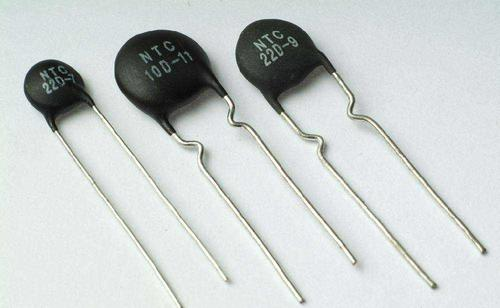NTC Thermal Resistor Introduction
Mar. 27, 2023

The introduction of NTC thermistor resistor, NTC (negaTive Temperature Coefficient) refers to the thermistor phenomenon and material with a negative temperature coefficient and the resistance decreases exponentially as the temperature rises.

The material is a semiconductor ceramic made of two or more metal oxides such as manganese, copper, silicon, cobalt, iron, and zinc, which are fully mixed, molded, and sintered. It can be made into a thermistor with a negative temperature coefficient (NTC), in which the resistivity and material constant vary with the material composition ratio, sintering atmosphere, sintering temperature and structural state.At present, non-oxide NTC thermistor materials represented by silicon carbide, tin selenide, and tantalum nitride are also available.

NTC thermal semiconducting ceramics are mostly oxide ceramics with a spinel structure or other structures, which have a negative temperature coefficient. The resistance value can be approximated as: RT0 is the resistance value at temperature T and T0, respectively, and Bn is the material constant. The resistivity of the ceramic grain itself changes due to temperature changes, which is determined by the characteristics of the semiconductor.

The development of NTC thermistor has a long phase. In 1834, scientists first discovered that silver sulfide has a negative temperature coefficient. In 1930, scientists discovered that cuprous oxide-copper oxide also has a negative temperature coefficient. It was successfully applied to the temperature compensation circuit of aeronautical instruments. Subsequently, due to the continuous development of crystal technology, the thermistor research made significant progress.

NTC thermistor was developed in 1960. NTC thermistor is widely used in temperature measurement, temperature control, temperature compensation and so on. The following describes an example application of temperature measurement. The temperature measurement range of NTC thermistor is generally -10 to +300°C, and it can also be used for temperature measurement in an environment of +300 to +1200°C. It can also be used at -200 to +10°C.

RT is the NTC resistor, R2 and R3 are the bridge balance resistance, R1 is the starting resistance, R4 is the full-scale resistance, the calibration meter head, also known as the calibration resistance, R7 and R8 and W Wei divider resistance, Provide a stable DC power supply for the bridge. R6 is connected in series with the meter head to correct the scale of the meter head and limit the current flowing through the meter head. R5 and the meter head are connected in parallel to protect the unbalanced bridge arm. Connect a thermal element RT as a temperature sensor probe.

Because the resistance of the thermistor changes with the temperature, the indicator of the meter connected between the diagonals of the bridge also changes accordingly. This is the working principle of the thermistor thermometer.

The accuracy of the thermistor thermometer can reach 0.1℃, and the time to ask can be as short as 10s or less. It is not only suitable for granary thermometers, but also for food storage, medicine and health, scientific farming, oceans, deep wells, and high altitudes. Temperature measurement of glaciers and other aspects.

Share: